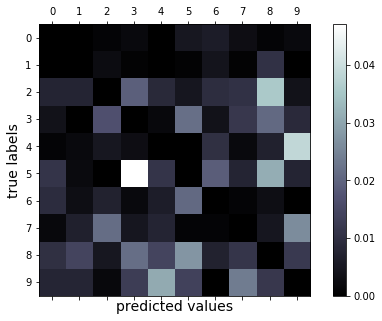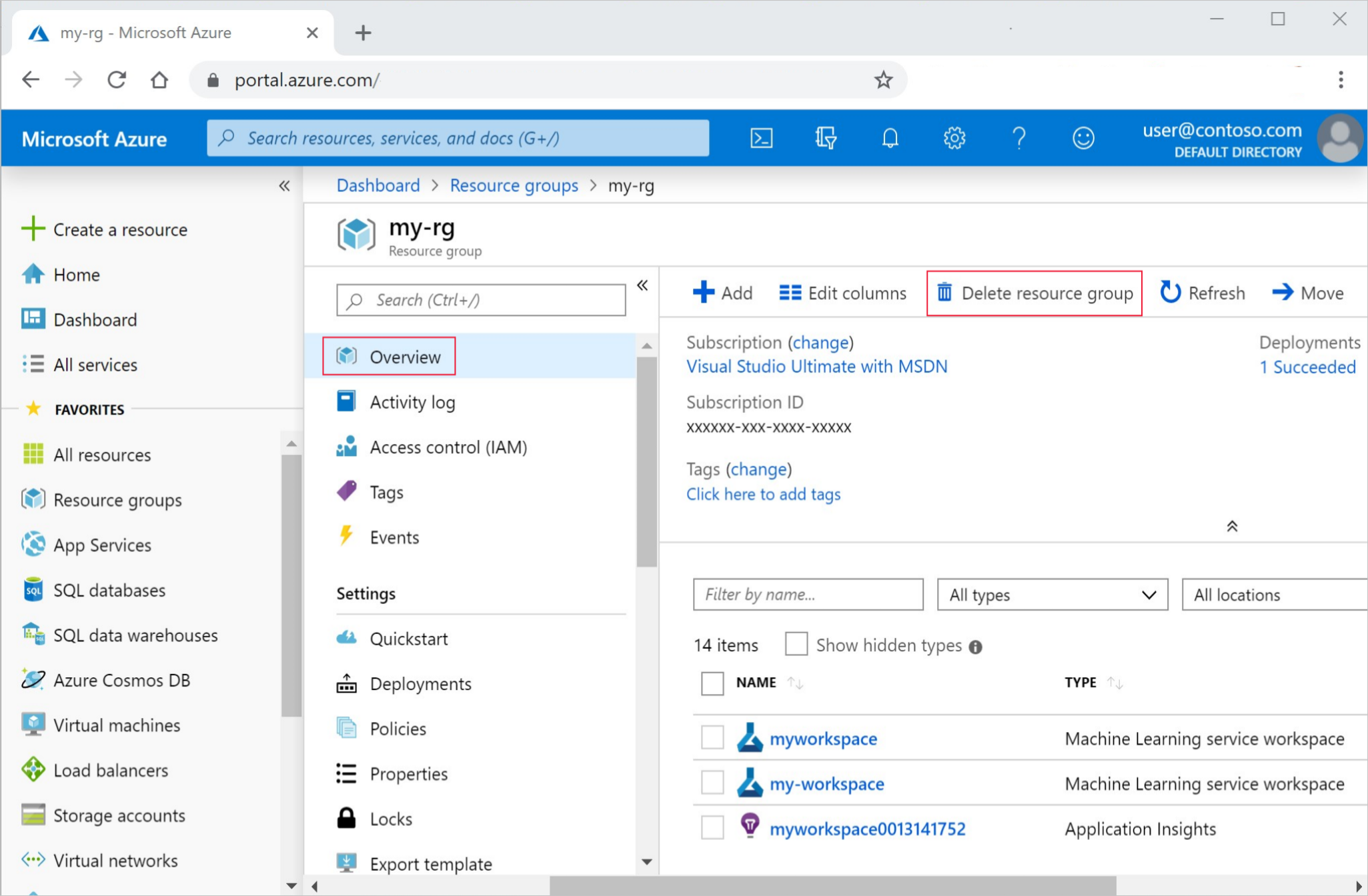# 教程：在 Azure 容器实例中部署映像分类模型

• 设置测试环境。
• 从工作区检索模型。
• 将模型部署到容器实例。
• 测试已部署的模型。

## 设置环境

### 导入包

``````%matplotlib inline
import numpy as np
import matplotlib.pyplot as plt

import azureml.core

# Display the core SDK version number
print("Azure ML SDK Version: ", azureml.core.VERSION)
``````

## 部署为 Web 服务

• 显示如何使用模型的评分脚本
• 要生成 ACI 的配置文件
• 之前已定型的模型

### 创建评分脚本

• `init()` 函数，它通常将模型加载到全局对象中。 此函数只能在 Docker 容器启动时运行一次。

• `run(input_data)` 函数，它使用模型来基于输入数据预测值。 运行的输入和输出通常使用 JSON 进行序列化和反序列化，但支持其他格式。

``````%%writefile score.py
import json
import numpy as np
import os
import pickle
import joblib

def init():
global model
# AZUREML_MODEL_DIR is an environment variable created during deployment.
# It is the path to the model folder (./azureml-models/\$MODEL_NAME/\$VERSION)
# For multiple models, it points to the folder containing all deployed models (./azureml-models)
model_path = os.path.join(os.getenv('AZUREML_MODEL_DIR'), 'sklearn_mnist_model.pkl')

def run(raw_data):
# make prediction
y_hat = model.predict(data)
# you can return any data type as long as it is JSON-serializable
return y_hat.tolist()
``````

### 创建配置文件

``````from azureml.core.webservice import AciWebservice

aciconfig = AciWebservice.deploy_configuration(cpu_cores=1,
memory_gb=1,
tags={"data": "MNIST",  "method" : "sklearn"},
description='Predict MNIST with sklearn')
``````

### 在 ACI 中部署

1. 使用训练过程中保存的环境 (`tutorial-env`) 创建包含模型所需依赖项的环境对象。
2. 使用以下项创建将模型部署为 Web 服务所需的推理配置：
• 评分文件 (`score.py`)
• 在上一步中创建的环境对象
3. 将模型部署到 ACI 容器。
4. 获取 Web 服务 HTTP 终结点。
``````%%time
from azureml.core.webservice import Webservice
from azureml.core.model import InferenceConfig
from azureml.core.environment import Environment
from azureml.core import Workspace
from azureml.core.model import Model

ws = Workspace.from_config()
model = Model(ws, 'sklearn_mnist')

myenv = Environment.get(workspace=ws, name="tutorial-env", version="1")
inference_config = InferenceConfig(entry_script="score.py", environment=myenv)

service = Model.deploy(workspace=ws,
name='sklearn-mnist-svc3',
models=[model],
inference_config=inference_config,
deployment_config=aciconfig)

service.wait_for_deployment(show_output=True)
``````

``````print(service.scoring_uri)
``````

## 测试模型

### 下载测试数据

``````import os
from azureml.core import Dataset
from azureml.opendatasets import MNIST

data_folder = os.path.join(os.getcwd(), 'data')
os.makedirs(data_folder, exist_ok=True)

mnist_file_dataset = MNIST.get_file_dataset()
``````

### 加载测试数据

``````from utils import load_data
import os
import glob

data_folder = os.path.join(os.getcwd(), 'data')
# note we also shrink the intensity values (X) from 0-255 to 0-1. This helps the neural network converge faster
X_test = load_data(glob.glob(os.path.join(data_folder,"**/t10k-images-idx3-ubyte.gz"), recursive=True), False) / 255.0
``````

### 预测测试数据

1. 将数据作为 JSON 数组发送到 ACI 中托管的 Web 服务。

2. 使用 SDK 的 `run` API 来调用服务。 还可以使用任何 HTTP 工具（如 curl）进行原始调用。

``````import json
test = json.dumps({"data": X_test.tolist()})
test = bytes(test, encoding='utf8')
y_hat = service.run(input_data=test)
``````

### 检查混淆矩阵

``````from sklearn.metrics import confusion_matrix

conf_mx = confusion_matrix(y_test, y_hat)
print(conf_mx)
print('Overall accuracy:', np.average(y_hat == y_test))
``````

``````[[ 960    0    1    2    1    5    6    3    1    1]
[   0 1112    3    1    0    1    5    1   12    0]
[   9    8  920   20   10    4   10   11   37    3]
[   4    0   17  921    2   21    4   12   20    9]
[   1    2    5    3  915    0   10    2    6   38]
[  10    2    0   41   10  770   17    7   28    7]
[   9    3    7    2    6   20  907    1    3    0]
[   2    7   22    5    8    1    1  950    5   27]
[  10   15    5   21   15   27    7   11  851   12]
[   7    8    2   13   32   13    0   24   12  898]]
Overall accuracy: 0.9204
``````

``````# normalize the diagonal cells so that they don't overpower the rest of the cells when visualized
row_sums = conf_mx.sum(axis=1, keepdims=True)
norm_conf_mx = conf_mx / row_sums
np.fill_diagonal(norm_conf_mx, 0)

fig = plt.figure(figsize=(8, 5))
cax = ax.matshow(norm_conf_mx, cmap=plt.cm.bone)
ticks = np.arange(0, 10, 1)
ax.set_xticks(ticks)
ax.set_yticks(ticks)
ax.set_xticklabels(ticks)
ax.set_yticklabels(ticks)
fig.colorbar(cax)
plt.ylabel('true labels', fontsize=14)
plt.xlabel('predicted values', fontsize=14)
plt.savefig('conf.png')
plt.show()
``````## 显示预测

1. 打印返回的预测并将其与输入映像一起绘制。 红色字体和反向图像（黑底白色）用于突出显示错误分类的样本。

``````import json

# find 30 random samples from test set
n = 30
sample_indices = np.random.permutation(X_test.shape)[0:n]

test_samples = json.dumps({"data": X_test[sample_indices].tolist()})
test_samples = bytes(test_samples, encoding='utf8')

# predict using the deployed model
result = service.run(input_data=test_samples)

# compare actual value vs. the predicted values:
i = 0
plt.figure(figsize = (20, 1))

for s in sample_indices:
plt.subplot(1, n, i + 1)
plt.axhline('')
plt.axvline('')

# use different color for misclassified sample
font_color = 'red' if y_test[s] != result[i] else 'black'
clr_map = plt.cm.gray if y_test[s] != result[i] else plt.cm.Greys

plt.text(x=10, y=-10, s=result[i], fontsize=18, color=font_color)
plt.imshow(X_test[s].reshape(28, 28), cmap=clr_map)

i = i + 1
plt.show()
``````

``````import requests

# send a random row from the test set to score
random_index = np.random.randint(0, len(X_test)-1)
input_data = "{\"data\": [" + str(list(X_test[random_index])) + "]}"

# for AKS deployment you'd need to the service key in the header as well
# api_key = service.get_key()
# headers = {'Content-Type':'application/json',  'Authorization':('Bearer '+ api_key)}

print("POST to url", service.scoring_uri)
#print("input data:", input_data)
print("label:", y_test[random_index])
print("prediction:", resp.text)
``````

## 清理资源

``````service.delete()
``````

1. 在 Azure 门户中，选择最左侧的“资源组” 。

2. 从列表中选择你创建的资源组。

3. 选择“删除资源组”4. 输入资源组名称。 然后选择“删除”。